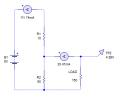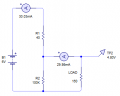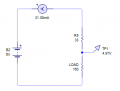# Voltage Dividers for voltage regulation

#### Transatlantic

Joined Feb 6, 2014
44
(I know there are better ways to create voltage regulators, but I'm going through the basics)

How do you determine the resistors used for a voltage divider?

Say I have a 6v supply and I want a 5v output, where my load draws 30ma. For this, the lower half of the divider would need to be 6 times the resistance of the upper half.

I've read that you want to keep the divider as 'Stiff' as possible. One way to do this is to keep the values low, this way, changes in the load won't affect Vout much. But not too low as to have the supplies internal resistance come into the picture. So I might use for example, a 60ohm and a 10ohm.The problem here is that it's not very efficient. I'm drawing 0.7w, where as my load is only using 0.15w. So I'm wondering how you calculate the optimal values for R1 and R2?

Here are my thoughts.

- The load has a resistance of 160ohms
- The circuit needs to be drawing around 30ma
- The lower half needs to be 6 times higher than the upper half

To achieve this, I can calculate it as a single resistor.

6v / 0.03ma = 200ohm
As the load resistor is 160ohm, that leaves 40ohm for R1.
To keep the lower parallel part at around 160ohms, I can set R2 to be something very high, like 100kThis is now more efficient. Instead of drawing 0.7w, it's now 0.18w

Is my reasoning/logic correct? .. is there a more accurate way to do this?

This raises another question for me though. Why bother with R2 in the first place? why not keep things simple and have R1 and RL in series?I assume the answer is do with the first circuit being better at handling changes in the load resistance, at the cost of excess power draw? So the circuit above would only ever work if the load had a very stable resistance.

#### dl324

Joined Mar 30, 2015
15,510
If you want to use a resistor divider, current in the divider needs to be at least 10X the load current. A 30mA load would require 300mA in the divider.

Total divider resistance R=V/I=6V/0.3A=20Ω
Vout = Vin*R2/(R1+R2)

Too much power wasted; use a more efficient method.

#### AnalogKid

Joined Aug 1, 2013
10,218
Say I have a 6v supply and I want a 5v output, where my load draws 30ma. For this, the lower half of the divider would need to be 6 times the resistance of the upper half.
Nope. 5 times.
So the circuit above would only ever work if the load had a very stable resistance.
Conceptually correct, except for the math error.

And as you have determined, the result is not "regulated". That is, one output characteristic is not (relatively) independent of the other. A voltage regulator holds the output voltage constant over a range of output current values. What you have is a voltage divider.

ak

Last edited:

#### WBahn

Joined Mar 31, 2012
28,171